﻿ Mathematical Study of Blood Flow in a Circular Tube of Varying Cross-section of Non-newtonian Biviscous Incompressible Fluid in the Permeable Wall

### Mathematical Study of Blood Flow in a Circular Tube of Varying Cross-section of Non-newtonian Bivisc...

Anil Kumar

Biomedical Science and Engineering

## Mathematical Study of Blood Flow in a Circular Tube of Varying Cross-section of Non-newtonian Biviscous Incompressible Fluid in the Permeable Wall

Department of Applied Science (Mathematics), Chandigarh Engineering College Landran Mohali Punjab

### Abstract

The present paper, consider a pulsatile fluid flow of blood in a circular tube with permeable wall of varying cross-section has been investigatation of non- Newtonian biviscous incompressible fluid. The governing equations are solved by perturbation scheme. The results are depicts of wall shear stress and pressure drop have been discussed and shown graphically of suction and injection.

• Anil Kumar. Mathematical Study of Blood Flow in a Circular Tube of Varying Cross-section of Non-newtonian Biviscous Incompressible Fluid in the Permeable Wall. Biomedical Science and Engineering. Vol. 5, No. 1, 2017, pp 1-4. http://pubs.sciepub.com/bse/5/1/1
• Kumar, Anil. "Mathematical Study of Blood Flow in a Circular Tube of Varying Cross-section of Non-newtonian Biviscous Incompressible Fluid in the Permeable Wall." Biomedical Science and Engineering 5.1 (2017): 1-4.
• Kumar, A. (2017). Mathematical Study of Blood Flow in a Circular Tube of Varying Cross-section of Non-newtonian Biviscous Incompressible Fluid in the Permeable Wall. Biomedical Science and Engineering, 5(1), 1-4.
• Kumar, Anil. "Mathematical Study of Blood Flow in a Circular Tube of Varying Cross-section of Non-newtonian Biviscous Incompressible Fluid in the Permeable Wall." Biomedical Science and Engineering 5, no. 1 (2017): 1-4.

 Import into BibTeX Import into EndNote Import into RefMan Import into RefWorks

1
Prev Next

### 1. Introduction

The control of the blood pressure has been possible by using porous effect in cases of cholesterol and related diseases. For describing the mechanics of red blood cell motion in narrow capillaries, we distinguish two situations according to the convenience with which the cells fit into the vessels. Womersley [1, 2] considered the oscillatory flow in a cylindrical tube with uniform cross-section. Lee and Fung  studied the flow of blood through an artery with an axisymmetric stenosis taking blood as a Newtonian fluid. Bitoun Bellet  studied pulsatile flow of blood with reference to stenosis in microcirculation. Radhakrishnamacharya et al.  and Prasad et al.  studied the pulsatile flow of blood in circular tubes of varying cross-section with Suction/injection. But the non- Newtonian property is not taken into consideration in these studies. Nakayama and Sawada  studied the flow of a non- Newtonian fluid through an axisymmetric stenosis numerically. The pulsatile flow of a non- Newtonian biviscous fluid through a tube with varying cross-section and non- permeable walls in presence of external magnetic field has been analysed by Elnaby et al. . Sanyal et al.  investigated the pulsatile flow of biviscous fluid through a tube of varying cross-section with suction/injection. But they considered no effect of slip velocity at the wall of the tube and so the effect of slip velocity has been neglected. Our main object in the present work is to study the pulsatile motion of blood in a circular tube of permeable wall and varying cross-section in presence of slip velocity at the tube wall. In this analysis, we assume that blood is a non- Newtonian biviscous fluid and the blood vessel is a straight, rigid circular tube of varying cross-section. Kumar at el.  founded computational technique for flow in blood vessels with porous effects and their using Galerkin finite element method. Gupta  investigated a performance and analysis of blood flow through carotid artery and their using finite element method. Gupta  made performance modeling and mechanical behaviour of blood vessel in the presence of magnetic effects and they are using finite difference method.

### 2. Mathematical Model

In the present communication of pulsatile motion for an incompressible non- Newtonian biviscous fluid in an axi-symmetric rigid circular tube of varying cross-section and permeable wall with slip flow is considered.

Then the radius of the tube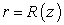is given by(1)

The governing equation of the pulsatile flow of an incompressible non- Newtonian fluid obeying biviscosity model axisymmetric circular artery are given by: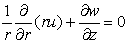(2)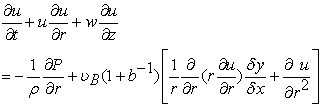(3)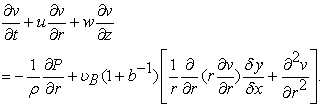(4)

The normal component of the fluid velocity at the tube wall is given by(5)

whereis the steady state suction/injection velocity,is the ratio of the amplitudes of the oscillatory and steady parts of the suction/injection velocity andis the frequency of the oscillation.

The non-dimensional form is given below:(6)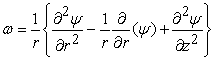(7)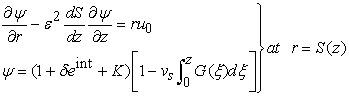(8)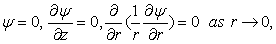(9)

where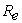is the Reynolds number of entrance flow,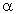is Womersley’s parameter,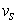is the leakage parameter and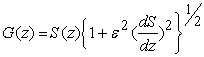and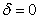and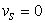.

### 3. Perturbation Technique

We assume that the pulsatile flow consists of the steady part and the oscillatory part of small amplitude of oscillation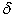such that the terms of the ordercan be neglected (ie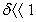) and the expression of vortices and stream function are given by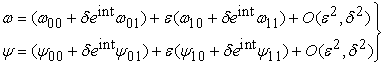(10)

The shear stress is given by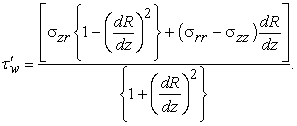Then using the boundary conditions at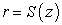and equations (6) and (7), we obtain the dimensionless wall shear stress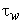are given by: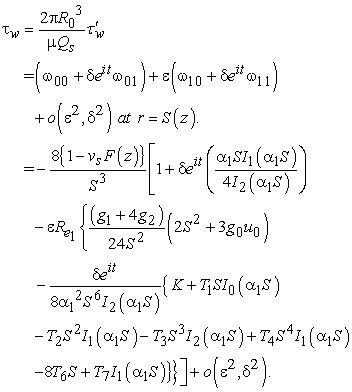(11)

The pressure drop are given by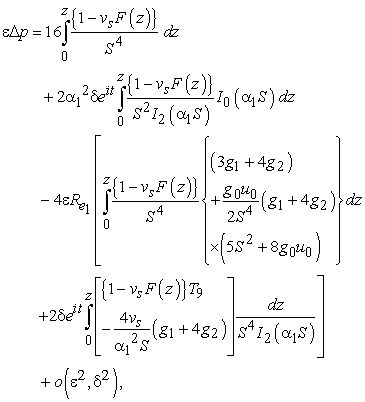(12)

where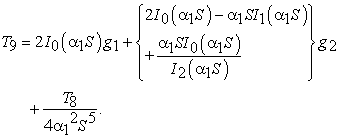### 4. Results and Discussion

The real part of dimensionless streamlinesis plotted for different values of slip parameter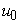, Reynolds numberand upper limit of apparent viscosity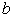in Figure 1 of the value ofdecreases in the converging region and increases in the diverging region of the tube. From Figure 2, Figure 3, it is seen that the similar results occur for a locally constricted tube. The effects of different parameters on the real part of dimensionless pressure drop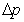are indicated graphically through Figure 2. And Figure 3 depicts that of thedecreases with increase inand increases with increase infor both suction and injection velocities.Download asVeiw figureFigures index
Figure 1. ψ vs z for sinusiodal tube with injection at the permeable wallDownload asVeiw figureFigures index
Figure 2. Δp vs z for sinusoidal tube with suction at the permeable wallDownload asVeiw figureFigures index
Figure 3. Δp vs z for sinusoidal tube with injection at the permeable wall

### 5. Conclusions and Applications

This investigation helps us to note that the influence of permeable parameter in the pressure drop is much significant and decreases rapidly with increases in slip parameter. It is also to be noted that this presentation help us to draw the flow characteristic of blood and the wall shear stress on the inner permeable wall of capillaries and small blood vessels where suction, injection and slip velocities arises and Reynolds number is very low. So, this investigation may be helpful in various fields of medical science.

### References

  Womersley, JR.. Method for the calculation of velocity, rate of flow and viscous drag in arteries when the pressure gradient is known, Journal of physiology, 127 (1955) 553-563.In article View Article  PubMed  Womersley, JR.. Oscillatory motion of a viscous liquid in a thin-walled elastic tube. I. The linear approximation for long waves, Phil. Mag., 46 (1955) 199-221.In article View Article  Lee, JS., Fung, YC.. Flow in locally constricted tubes at low Reynolds number, ASME J. Appl. Mech., 37 (1970) 9-16.In article View Article  Rao, AR., Devanathan, R.. Pulsatile flow in tubes of varying cross-section, Z.A.M.P., 24 (1973) 203-213.In article View Article  Schneck, DJ., Ostrach, S.. Pulsatile blood flow in a channel of small exponential divergence-I. The linear approximation for low mean Reynolds number, Journal of Fluids Engineering., 16 (1975) 353-360.In article View Article  Bitoun, JP., Bellet, D.. Blood flow through a stenosis in micro-circulation, Biorheology, 23 (1986) 51-61.In article PubMed  Manton, MJ.. Low Reynolds number flow in slowly varying axisymmetric tubes, Journal of Fluid Mech., 49 (1971) 451-459.In article View Article  Radhakrishnamacharya, G., Chandra, P., Kaimal, MR.. A hydro dynamical study of flow in renal tubule, Bull. Math. Biol., 43 (1981) 151-163.In article View Article  PubMed  Chandra, P., Prasad, JS.. Pulsatile flow in circular tubes of varying cross-section with suction/injunction, J. Austral. Math. Soc., 35 (1994) 336-381.In article  Chow, JCF.. Blood flow theory, effective viscosity and effects of particle distribution, Bull. Math. Biol., 37 (1975) 472-488.In article View Article  Hill, CD., Bedford, A.. A model for erythrocyte sedimentation, Biorheology, 18 (1981) 255.In article PubMed  Srivastava, LM., Agarwal, RP.. Oscillating flow of a conducting fluid with a suspension of spheical particles, J. Appl. Mech., 47 (1980) 196.In article View Article  Nakayama, M., Sawada, TJ.. Numerical study on the flow of a non- Newtonian fluid through an axisymmetric stenosis, Biomech. Eng., 110 (1988) 137.In article View Article  Elnaby, MA., Eldabe, NT M., Abou Zied, MY., Sanyal, DC.: Mathematical analysis on M.H.D. pulsatile flow of a non- Newtonian fluid through a tube with varying cross-section, J. of Inst. of Math. and Comp. Sci., 20 (2007) 29-42.In article  Sanyal, DC., Das, K., Debnath, S.. Pulsatile flow of biviscous fluid through a tube of varying cross-section, International Journal of computational Intelligence and Healthcare Informatics, 1 (2008) 1-8.In article  Raoufpanah, A., Rad, M., Borujerdi AN.. Effects of slip condition on the characteristic of flow in ice melting process, IJE Transactions B: Applications 18 (2005), 1-9.In article  Das.K.. Heat transfer peristaltic transport with slip condition in an asymmetric porous channel, IJE Transactions B: Applications, 24(3)(2011), 293-307.In article  Kumar, Anil, Varshney, C.L. and Sharma, G.C. (2005) Computational technique for flow in blood vessels with porous effects. Applied Mathematics and Mechanics, (2005)26, 63-72.In article View Article  Anil Kumar Gupta (2011). Performance and analysis of blood flow through carotid artery, International Journal of Engineering and Business Management vol. 3(4) pp 1-6.In article View Article  Anil Kumar Gupta (2013). Performance modeling and mechanical behaviour of blood vessel in the presence of magnetic effects, African Journal of Basic & Applied Sciences 5 (3): 149-155.In article